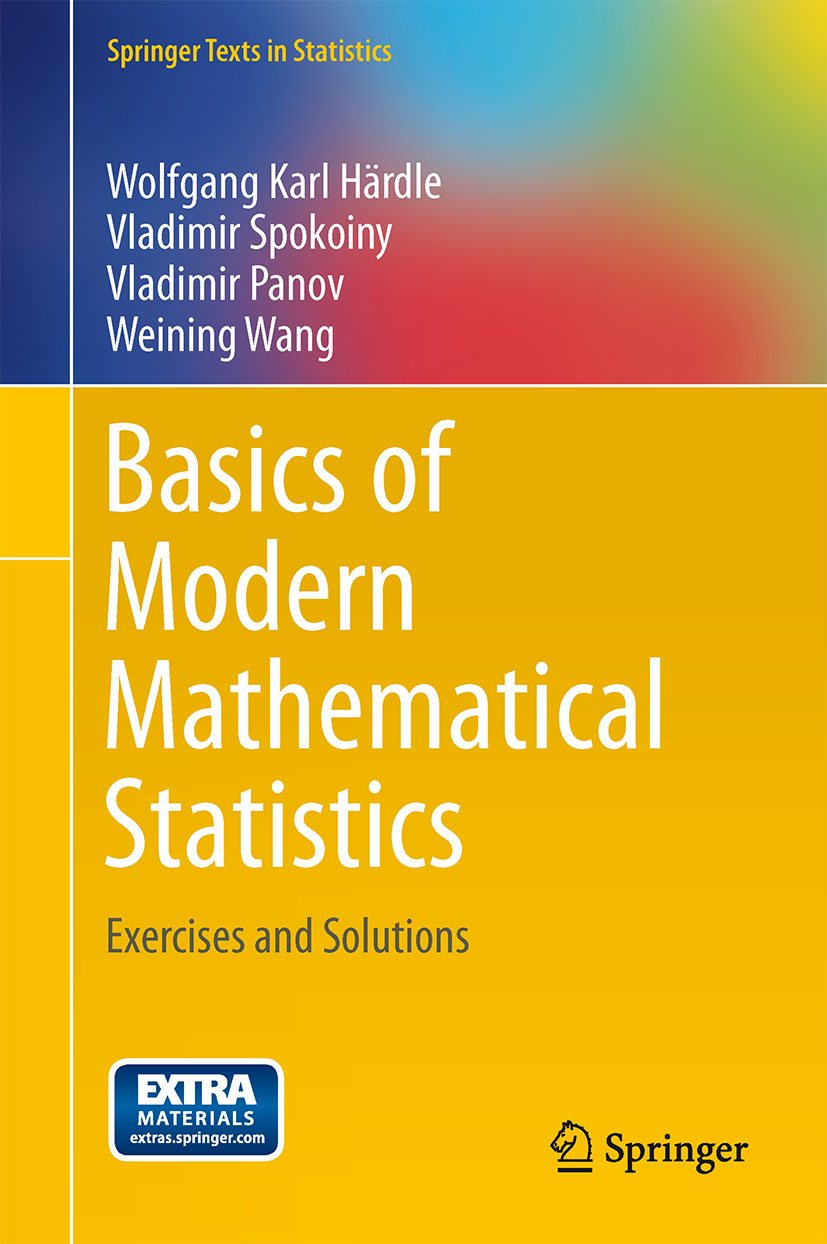• A
• A
• A
• ABC
• ABC
• ABC
• А
• А
• А
• А
• А
Regular version of the site

## Basics of modern mathematical statistics: exercises and solutions

Iss. XXV. Heidelberg: Springer, 2013.
Härdle W., Spokoiny V., Panov V., Wang W.
• ​Presents numerous exercises with solutions to help the reader better understand different aspects of modern statistics
• Applications with R and Matlab code show how to practically use the methods
• Includes numerous explanations and tips on how to apply modern statistical methods ​

The complexity of today’s statistical data calls for modern mathematical tools. Many fields of science make use of mathematical statistics and require continuous updating on statistical technologies. Practice makes perfect, since mastering the tools makes them applicable. Our book of exercises and solutions offers a wide range of applications and numerical solutions based on R. In modern mathematical statistics, the purpose is to provide statistics students with a number of basic exercises and also an understanding of how the theory can be applied to real-world problems. The application aspect is also quite important, as most previous exercise books are mostly on theoretical derivations. Also we add some problems from topics often encountered in recent research papers. The book was written for statistics students with one or two years of coursework in mathematical statistics and probability, professors who hold courses in mathematical statistics, and researchers in other fields who would like to do some exercises on math statistics.